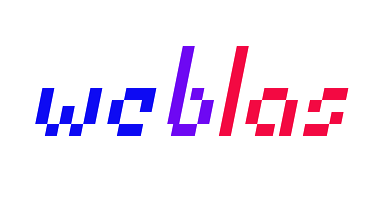GPU accelerated Javascript. Numerical computing in your browser with performance comparable to native.

Currently includes hundreds of unit tests, which verify correctness on hundreds of millions of data points.

# Operations

Our focus is on numerical operations useful for neural networks and machine learning. So far, we've got 32-bit versions of each of these:

• sscal - Matrix (and Vector) Scale (with addition)
• sgemm - Matrix Multiply
• sdwns - Matrix (and Image) Downsample (for Max Pooling)
• sclmp - Matrix clamp (for ReLU)

Don't see what you need? Give a 👍 to an existing issue or create a new one!

# Usage

First, include the `weblas.js` file (from a release or the `dist` directory).

``````<script type="text/javascript" src="weblas.js"></script>
``````

Then use it like this.

``````<script>

// create Matrices as arrays
var height_A = 1024, width_A = 1024,
height_B = 1024, width_B = 1024;

var A = new Float32Array(height_A * width_A);
var B = new Float32Array(height_B * width_B);

// fill A and B with science

var M = height_A,
N = width_B,
K = height_B; // must match width_A

var alpha = 1.0;
var beta = 0.0;
var C = new Float32Array(width_B)      // specialized for neural net bias calculation

// result will contain matrix multiply of A x B (times alpha)
result = weblas.sgemm(M, N, K, alpha, A, B, beta, C);

</script>
``````

## Pipeline Mode

Pipeline mode gives (sometimes very large) increases in performance by leaving data in GPU memory. A demo illustrating performance on a deep neural net can be found here.

Here's a basic example:

``````// create Tensor containers for interacting directly with GPU memory
var t0 = new weblas.pipeline.Tensor([M, K], data0);
// second matrix must be transposed
var t1 = new weblas.pipeline.Tensor([N, K], weblas.util.transpose(K, N, data1));
var t2 = new weblas.pipeline.Tensor([1, N], data2);
var alpha = 1.0;
var beta = 0.5;

/* NOTE: pipeline.sgemm takes a transpose matrix in the
second slot (t1 here)
(this requirement allows for improved performance)
*/
var t3 = weblas.pipeline.sgemm(alpha, t0, t1, beta, t2);

// result is a Float32Array
var result = t3.transfer();
``````

# Testing

Unit tests and benchmarks both require `browserify` and `testling`.

Install with:

``````npm install -g browserify
npm install -g testling
``````

## Unit Tests

All operations have unit test coverage. Unit tests use data generated outside the browser (to verify correctness). Generating the data requires `python` and the modules in `requirements.txt`.

With `pip` installed run:

``````pip install -r requirements.txt
``````

Then, to generate the data, run:

``````npm run data
``````

Then, run the unit tests with:

``````npm test
``````

### OS Setup

If the tests won't run, try this (it restores the default npm browser setting)

#### OSX

``````npm config set browser open
``````

#### Linux

``````npm config set browser xdg-open
``````

#### Windows

``````npm config set browser start
``````

## Benchmarks

After installing `browserify` and `testling`, run the benchmarks with:

``````npm run benchmark
``````

### results

[email protected]

``````TAP version 13
ok 1 sgemm: 128x128 . 128x128
# 1.032 GFlops/sec  ±3.71%  n = 50 µ = 4ms
ok 2 sgemm: 128x256 . 256x128
# 1.745 GFlops/sec  ±2.89%  n = 44 µ = 5ms
ok 3 sgemm: 256x256 . 256x256
# 5.061 GFlops/sec  ±2.89%  n = 42 µ = 7ms
ok 4 sgemm: 512x256 . 256x512
# 15.454 GFlops/sec  ±3.86%  n = 51 µ = 9ms
ok 5 sgemm: 256x512 . 512x256
# 10.262 GFlops/sec  ±2.76%  n = 47 µ = 7ms
ok 6 sgemm: 512x512 . 512x512
# 22.231 GFlops/sec  ±3.54%  n = 50 µ = 12ms
ok 7 sgemm: 513x513 . 513x513
# 14.474 GFlops/sec  ±4.51%  n = 43 µ = 19ms
ok 8 sgemm: 1024x512 . 512x1024
# 41.859 GFlops/sec  ±3.38%  n = 43 µ = 26ms
ok 9 sgemm: 512x1024 . 1024x512
# 31.353 GFlops/sec  ±2.60%  n = 46 µ = 17ms
ok 10 sgemm: 1024x1024 . 1024x1024
# 45.545 GFlops/sec  ±3.99%  n = 31 µ = 47ms
ok 11 sgemm: 2048x2048 . 2048x2048
# 62.159 GFlops/sec  ±28.88%  n = 13 µ = 276ms

1..11
# tests 11
# pass  11

# ok
``````

# Donations

Want to see more happen here? Contribute onGet A Weekly Email With Trending Projects For These Topics
No Spam. Unsubscribe easily at any time.
Javascript (1,156,149
Testing (93,349
Pipeline (15,606
Benchmark (11,745
Matrix (10,866
Gpu (9,489
Blas (877